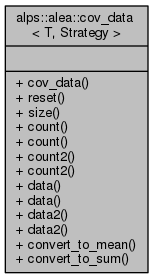ALPSCore reference
alps::alea::cov_data< T, Strategy > Class Template Reference

#include <convert.hpp>

Collaboration diagram for alps::alea::cov_data< T, Strategy >:## Public Types

typedef bind< Strategy, T >::value_type value_type

typedef bind< Strategy, T >::cov_type cov_type

typedef eigen< cov_type >::matrix cov_matrix_type

## Public Member Functions

cov_data (size_t size)

void reset ()

size_t size () const

size_t count () const

size_t & count ()

double count2 () const

double & count2 ()

const column< value_type > & data () const

column< value_type > & data ()

const cov_matrix_typedata2 () const

cov_matrix_typedata2 ()

void convert_to_mean ()

void convert_to_sum ()

## Friends

class cov_acc< T, Strategy >

class cov_result< T, Strategy >

void serialize (serializer &, const std::string &, const cov_result< T, Strategy > &)

void deserialize (deserializer &, const std::string &, cov_result< T, Strategy > &)

## Detailed Description

### template<typename T, typename Strategy = circular_var> class alps::alea::cov_data< T, Strategy >

Data for covariance accumulation.

As with mean_acc, this class is basically a "union"-like structure, which for a data series (X, ... X[count_-1]) either represents the sum of X[i] and the sum of X[i]*X[j] (sum state) or the sample mean and sample covariance of X (mean state).

Definition at line 20 of file convert.hpp.

## Member Typedef Documentation

template<typename T, typename Strategy = circular_var>
 typedef eigen::matrix alps::alea::cov_data< T, Strategy >::cov_matrix_type

Definition at line 54 of file covariance.hpp.

template<typename T, typename Strategy = circular_var>
 typedef bind::cov_type alps::alea::cov_data< T, Strategy >::cov_type

Definition at line 53 of file covariance.hpp.

template<typename T, typename Strategy = circular_var>
 typedef bind::value_type alps::alea::cov_data< T, Strategy >::value_type

Definition at line 52 of file covariance.hpp.

## Constructor & Destructor Documentation

template<typename T , typename Str >
 alps::alea::cov_data< T, Str >::cov_data ( size_t size )

Definition at line 16 of file covariance.cpp.

## Member Function Documentation

template<typename T , typename Str >
 void alps::alea::cov_data< T, Str >::convert_to_mean ( )

Definition at line 33 of file covariance.cpp.

template<typename T , typename Str >
 void alps::alea::cov_data< T, Str >::convert_to_sum ( )

Definition at line 50 of file covariance.cpp.

template<typename T, typename Strategy = circular_var>
 size_t alps::alea::cov_data< T, Strategy >::count ( ) const
inline

Returns sample size, i.e., number of accumulated data points

Definition at line 66 of file covariance.hpp.

template<typename T, typename Strategy = circular_var>
 size_t& alps::alea::cov_data< T, Strategy >::count ( )
inline

Returns sample size, i.e., number of accumulated data points

Definition at line 69 of file covariance.hpp.

template<typename T, typename Strategy = circular_var>
 double alps::alea::cov_data< T, Strategy >::count2 ( ) const
inline

Returns sum of squared weights

Definition at line 72 of file covariance.hpp.

template<typename T, typename Strategy = circular_var>
 double& alps::alea::cov_data< T, Strategy >::count2 ( )
inline

Returns sum of squared weights

Definition at line 75 of file covariance.hpp.

template<typename T, typename Strategy = circular_var>
 const column& alps::alea::cov_data< T, Strategy >::data ( ) const
inline

Definition at line 77 of file covariance.hpp.

template<typename T, typename Strategy = circular_var>
 column& alps::alea::cov_data< T, Strategy >::data ( )
inline

Definition at line 79 of file covariance.hpp.

template<typename T, typename Strategy = circular_var>
 const cov_matrix_type& alps::alea::cov_data< T, Strategy >::data2 ( ) const
inline

Definition at line 81 of file covariance.hpp.

template<typename T, typename Strategy = circular_var>
 cov_matrix_type& alps::alea::cov_data< T, Strategy >::data2 ( )
inline

Definition at line 83 of file covariance.hpp.

template<typename T , typename Str >
 void alps::alea::cov_data< T, Str >::reset ( )

Re-allocate and thus clear all accumulated data

Definition at line 24 of file covariance.cpp.

template<typename T, typename Strategy = circular_var>
 size_t alps::alea::cov_data< T, Strategy >::size ( ) const
inline

Number of components of the random vector (e.g., size of mean)

Definition at line 63 of file covariance.hpp.

## Friends And Related Function Documentation

template<typename T, typename Strategy = circular_var>
 friend class cov_acc< T, Strategy >
friend

Definition at line 95 of file covariance.hpp.

template<typename T, typename Strategy = circular_var>
 friend class cov_result< T, Strategy >
friend

Definition at line 96 of file covariance.hpp.

template<typename T, typename Strategy = circular_var>
 void deserialize ( deserializer & , const std::string & , cov_result< T, Strategy > & )
friend
template<typename T, typename Strategy = circular_var>
 void serialize ( serializer & , const std::string & , const cov_result< T, Strategy > & )
friend

The documentation for this class was generated from the following files: